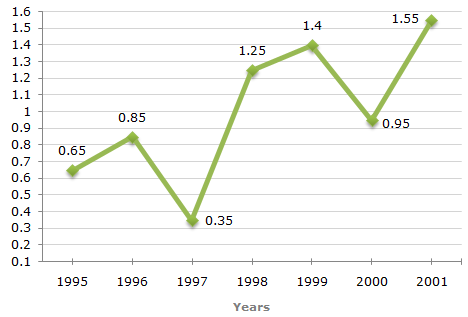# Data Interpretation - Line Charts

Exercise : Line Charts - Line Chart 2
Directions to Solve

The following line graph gives the ratio of the amounts of imports by a company to the amount of exports from that company over the period from 1995 to 2001.

Ratio of Value of Imports to Exports by a Company Over the Years.1.
If the imports in 1998 was Rs. 250 crores and the total exports in the years 1998 and 1999 together was Rs. 500 crores, then the imports in 1999 was ?
Rs. 250 crores
Rs. 300 crores
Rs. 357 crores
Rs. 420 crores
Explanation:

The ratio of imports to exports for the years 1998 and 1999 are 1.25 and 1.40 respectively.

Let the exports in the year 1998 = Rs. x crores.

Then, the exports in the year 1999 = Rs. (500 - x) crores.1.25 = 250x = 250 = 200       [Using ratio for 1998] x 1.25

Thus, the exports in the year 1999 = Rs. (500 - 200) crores = Rs. 300 crores.

Let the imports in the year 1999 = Rs. y crores.

 Then, 1.40 = yy = (300 x 1.40) = 420. 300Imports in the year 1999 = Rs. 420 crores.

2.
The imports were minimum proportionate to the exports of the company in the year ?
1995
1996
1997
2000
Explanation:

The imports are minimum proportionate to the exports implies that the ratio of the value of imports to exports has the minimum value.

Now, this ratio has a minimum value 0.35 in 1997, i.e., the imports are minimum proportionate to the exports in 1997.

3.
What was the percentage increase in imports from 1997 to 1998 ?
72
56
28
Explanation:

The graph gives only the ratio of imports to exports for different years. To find the percentage increase in imports from 1997 to 1998, we require more details such as the value of imports or exports during these years.

4.
If the imports of the company in 1996 was Rs. 272 crores, the exports from the company in 1996 was ?
Rs. 370 crores
Rs. 320 crores
Rs. 280 crores
Rs. 275 crores
Explanation:

Ratio of imports to exports in the year 1996 = 0.85.

Let the exports in 1996 = Rs. x crores.

 Then, 272 = 0.85x = 272 = 320. x 0.85Exports in 1996 = Rs. 320 crores.

5.
In how many of the given years were the exports more than the imports ?
1
2
3
4